Project

# Build Your Own Negative Voltage Generator

June 07, 2018 by Robin Mitchell

## Everyone needs a negative voltage rail eventually, but most only have a single rail supply. This project will show you how to build a negative voltage generator that runs off a single rail supply!

Everyone needs a negative voltage rail eventually, but most only have a single rail supply. This project will show you how to build a negative voltage generator that runs off a single rail supply!

### The Need for a Negative Rail

Many hobbyists will have come across an op-amp circuit that uses a dual rail supply. In order to provide the dual rails power, two power supplies can be used but many do not have access to such equipment or the design needs to be portable and thus two individual power supplies may not be ideal. One solution is to split the incoming power supply and create a virtual ground between two resistors in a potential divider.

However, this solution has some issues. Firstly, the current sinking / sourcing capability of the virtual ground is limited and, secondly, the supply voltage has been divided by 2 (for example, a 9V battery could become ±4.5V but 4.5V is the maximum achievable voltage).

But using an oscillator, some diodes, and a clever arrangement of capacitors, we can exploit an effect called capacitive coupling whereby a negative voltage near equal to the supply voltage can be generated... from a single rail supply!

### Some Capacitor Theory

Capacitive coupling is an effect where a capacitor “attempts” to keep the voltage across its terminals constant. For example, if both sides of a capacitor are at 0V and plate 1 is suddenly brought to 10V, the voltage on the other plate will immediately begin to rise to 10V. The same goes for the opposite: If the first plate is brought from 10V to 0V, then the other plate will attempt to drop by 10V to keep the voltage across the capacitor constant.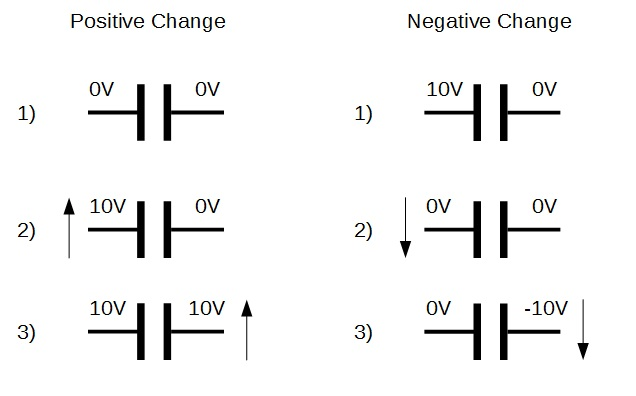Notice how the negative change gave a -10V when the left plate was charged to 10V and the other plate kept to 0V. This is the sequence that will be exploited to generate the negative voltage.

But how do we charge the left side to 10V without the other plate also charging to 10V? Remember that when you get the first plate to 10V the other side will also try to get to that voltage. This is where diodes come in!

### Diodes - Removing Positive Voltage

Remember how diodes only conduct in one direction? Well, if we use a simple (but clever) arrangement with a capacitor and two diodes, we can generate a negative voltage on a capacitor plate!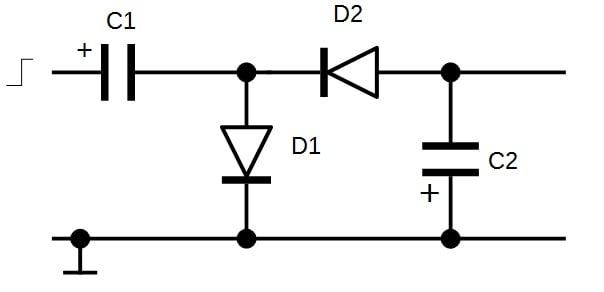As the input steps up from 0V to 10V, the negative plate on C1 will also try to match due to capacitive coupling. However, once the plate reaches 0.7V, D1 conducts and this prevents the plate voltage from getting higher than 0.7V. Now with the positive plate at 10V and the negative plate at 0.7V, the potential difference across the plate is 10 – 0.7 = 9.3V. When the positive plate drops rapidly from 10V to 0V, this 9.3V potential difference must be maintained due to coupling. Therefore, the negative plate drops to -9.3V. D1 cannot conduct as it is reversed biased, so this negative voltage remains on the capacitor.

D2, however, is now conducting because the anode (connected to C2 negative plate), is at a higher potential than the cathode (connected to the -9.3V). Therefore, the voltage at the negative plate of C2 will reduce until D2 stops conducting. D2 will stop conducting once the voltage drop across it becomes less than 0.7V. This will happen once the negative plate of C2 becomes -9.3 - -0.7 = -8.6V.

But if an external device attempts to use the negative voltage, it would very quickly be used up! This is because of the relatively small sizes of C1 and C2 as well as the current limits of the circuit driver. The solution is to connect an oscillator to the input so that a negative voltage is constantly being generated at C1, which keeps C2 topped up.

C2 is used as a decoupler for external devices using the negative voltage to try and keep the value as constant as possible. For our project, C1 will be a 10uF capacitor and C2 will be a 100uF capacitor.

### The Oscillator

The oscillator can be anything from a 555 timer to a microcontroller, but remember that the negative voltage will be equal to the oscillator's max voltage – min voltage. In this project, we will look at using two different oscillators: a 555 astable and a 74HC14 Schmitt trigger-based oscillator.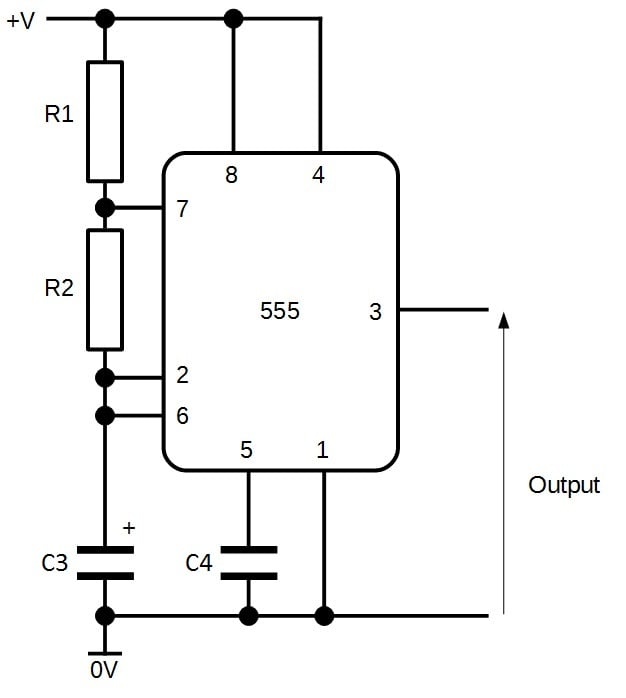##### 555 astable oscillator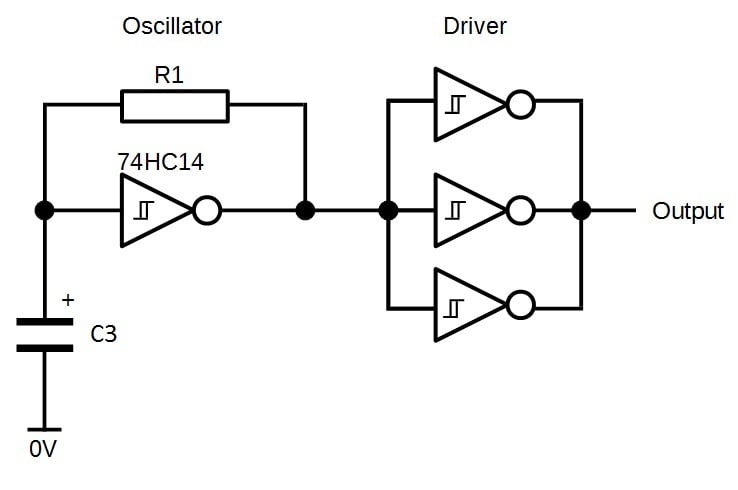### Bill Of Materials

BOM - Diode Pump Circuit

 Component Identifier Quantity Diode (Generic Silicon) D1, D2 2 10uF Electrolytic Capacitor C1 1 100uF Electrolytic Capacitor C2 1

BOM - 555 Oscillator

 Component Identifier Quantity 555 Timer IC 555 1 5.6kΩ Resistor R1 1 47kΩ Resistor R2 1 100nF Capacitor C4 1 10nF Capacitor C3 1 Assorted Wires - - Breadboard - 1

BOM - 74HC14 Oscillator

 Component Identifier Quantity 74HC14 IC (DIP) 74HC14 1 5.6kΩ Resistor R1 1 10nF Capacitor C3 1 Assorted Wires - - Breadboard - 1

Creating the circuit is very easy and requires at most an hour. Start with the oscillator and then build the capacitor pump circuit. As the 74HC14 oscillator only has one capacitor and resistor, the values are those listed in the BOM. The 555 timer uses a 5.6kΩ resistor for R1, a 47kΩ resistor for R2, 10nF capacitor for C1, and 100nF capacitor for C2.

If you have an oscilloscope, you can confirm that the oscillator works by probing the output. If you only have a multimeter, then you can measure the output using a DC voltage reading. There is a good chance that the circuit is oscillating if the reading is approximately half of the supply. For example, if you power your circuit from a 9V supply and the circuit is oscillating, then the output will read around 4.5V. This happens because the voltmeter takes multiple readings quickly and produces an average value. As the output is on for half the time and off for the other, half the average value is half of the max value.

### The Complete Circuits

555 driven negative voltage generator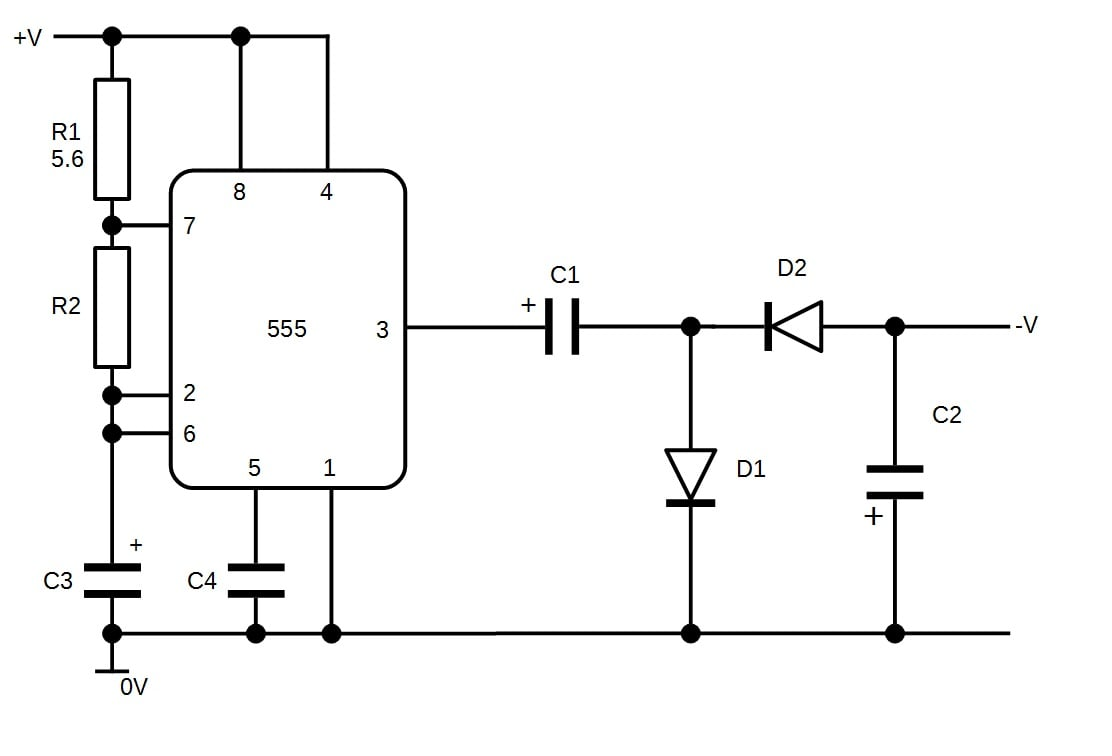74HC14 driven negative voltage generator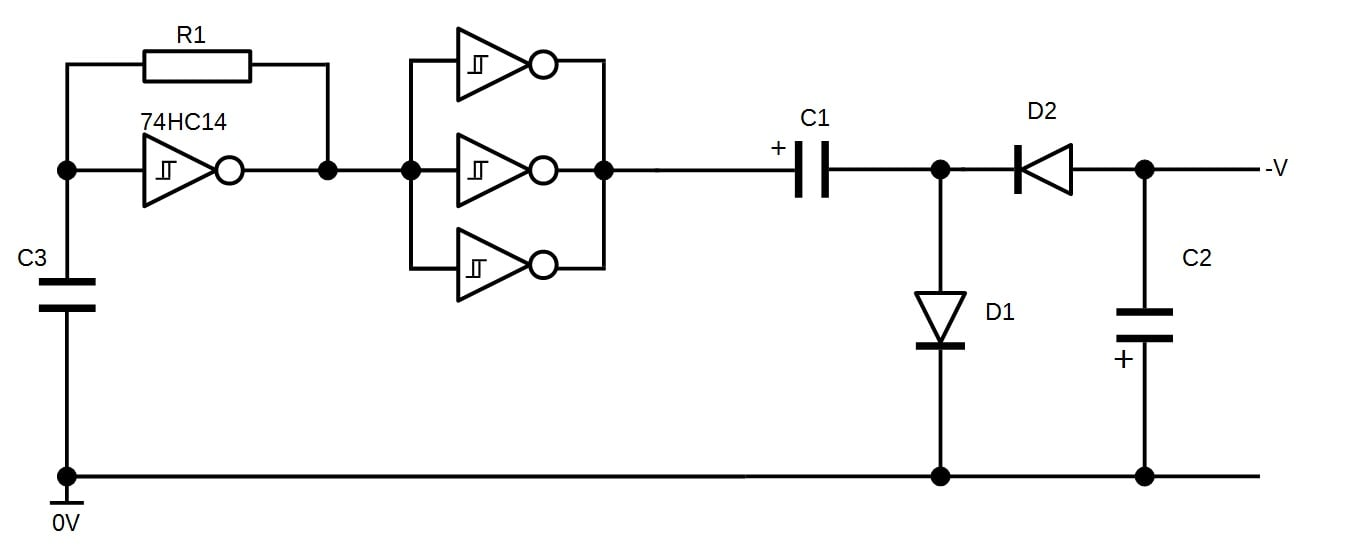### The Final Circuit

Here is the negative voltage generator using the 555 oscillator where the output is the blue wire on the right.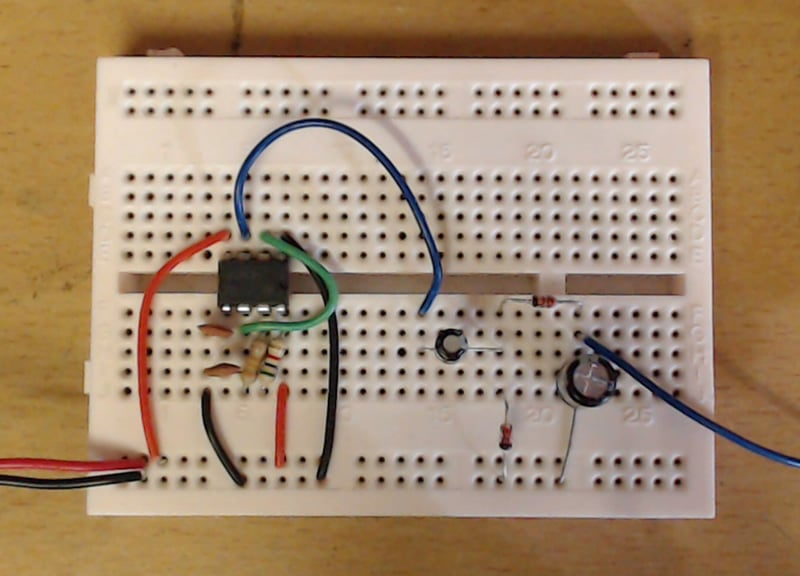Here is the output from the negative voltage generator. Note how the voltage is approximately -7.2V because of the double diode drop (1.4V), and the supply was not a perfect 9V but closer to 8.5V.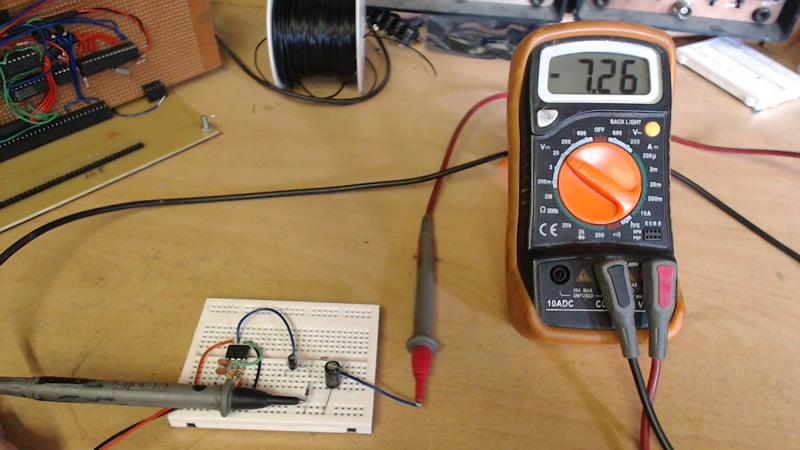Here is the construction using the 74HC14 oscillator: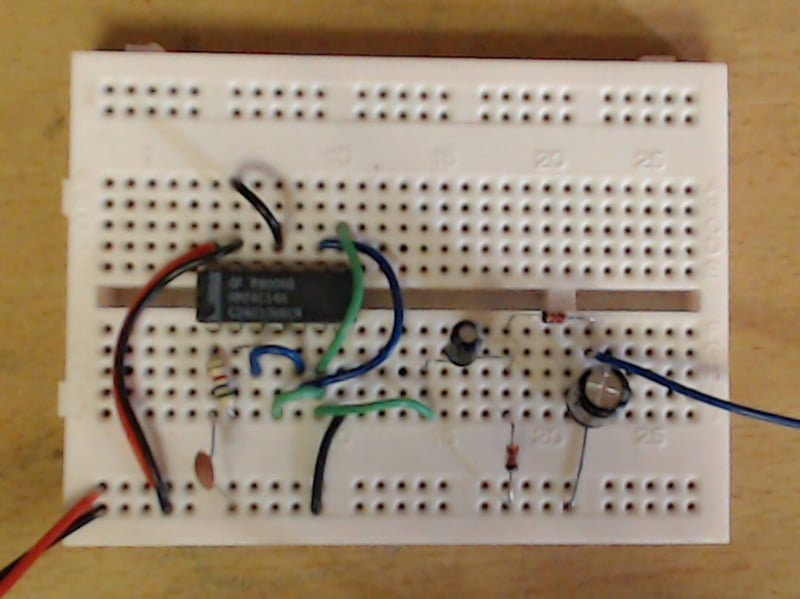The output here is -7.95V and is higher than the 555 timer circuit because the 74HC14 uses the excess gates to create a driver. This driver can source and sink more current than the 555's output and thus produce a larger negative voltage. Remember to connect all the unused inputs to ground on CMOS chips!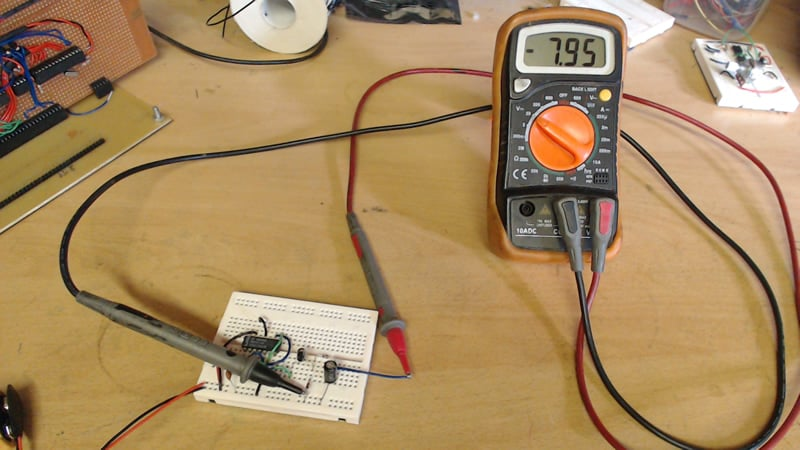### NVG Capabilities

This negative voltage generator can only source a small amount of current. If a regulator is used on the output (such as a 7905), don't expect to get more than 4mA ~ 5mA. But if left unregulated (such as in opamp projects), you can source up to 20mA before the output voltage begins to drop significantly. When using this generator in projects, try to keep the current draw from devices as small as possible so that the negative rail retains its voltage value.

The circuit can be made more efficient as well as increasing the output voltage by using Schottky diodes instead of generic silicon. This is because Schottky diodes have a smaller forward voltage drop (down to 0.1V), which results in a larger negative voltage.

### Summary

Now that you have a negative voltage generator circuit what can you do?

• Build a single rail dual supply : Using regulators and filtering components, you could make a dual rail supply that takes in a battery voltage or a wall wart adapter and converts it into a 5V and a -5V. If you use a large enough input voltage, you could also make a 12V and -12V line.
• Op-Amp Projects : Many op-amp projects (such as synthesizers) require the use of a negative rail and now you got one!
• Audio Projects : Audio components such as speakers and piezoelectric discs work better when they use both positive and negative voltages. This is because the negative voltage deforms the mechanism the opposite way the positive voltage deforms the mechanism. Therefore, the produced sound is much louder and clearer.
• Portable Dual Rail : If you have a system where weight needs to be reduced (such as an RC plan) that requires the use of a negative voltage, this circuit can be used instead of having two separate supplies, which would be heavy

Note : This project will be used in the up-coming series on DIY synthesizers so make sure you get one built soon!

Give this project a try for yourself! Get the BOM.• P
Paul Rautenbach June 17, 2016

Nice simple circuit. Thanks.

Like.
•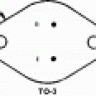to3metalcan June 17, 2016

I was going to comment that it’s odd that commercial power supplies don’t ever seem to be made this way, but then I realized that most switching power supplies ARE doing this, they’re just also doing it for the positive rail, too!

Like.
• M
mjaa June 21, 2016

Great article. SImple, educational and practical for hobbyists. Thank you!

Like.